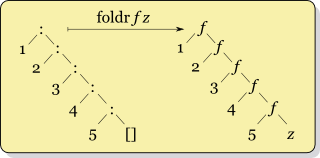# Fold and Traverse

## Introduction

Folding is the act of reducing a structure to a single value.

We can see them as consumers or `comonads`.

## foldl/foldr

``````foldr :: (a -> b -> b) -> b -> [a] -> b
foldr f v [] = v
foldr f v (x:xs) = f x (foldr f v xs)

foldl :: (s -> a -> s) -> s -> [a] -> s
foldl  f v []     =  v
foldl  f v (x:xs) =  foldl f (f v x) xs``````

Both functions take 3 arguments: - a combining function 'f' - a default value 'v' - the data to be folded.

The default value 'v' deals with the empty element of the list []. For non empty list, `foldl1/foldr1` is best suited.

### foldr

You can think of `foldr` non-recursively as simultaneously replacing each (:) in a list by a given function, and [] by a given value:

``foldr (-) 0 (1:2:3:[]) = (1 - ( 2 - (3 - 0))) = (1 - (2 - 3) = (1 - ( -1 ) = 2```Foldr` is handy if `f` is not strict in both arguments. That way we can rely on laziness to stop the recursion (or build an infinite list). `Map` for instance has to use `foldr` to maintain its laziness capabilities:

``````map = foldr (\x ys -> f x : ys) []
-- or map' = foldr ((:) . f) []

-- of course it can also be defined with recursion only
map' :: (a -> b) -> [a] -> [b]
map' _ [] = []
map' f (x:xs) = f x : (map f xs)

-- ex
takeWhile (< 12) \$ map (*2) [1..]``````

### foldl

On the other hand, when the whole list needs to be traversed (`sum` or `reverse` are two examples), `foldl'` is actually more efficient in term of memory space.

``````foldl (-) 0 (1:2:3:[]) = (0 - 1) - 2 - 3 = -6

reverse = foldl' (flip (:)) []``````

The strict version `List.foldl'` should always be used instead of the foldl from Prelude. The `Foldable` type class comes with `foldl` defined strictly.## Foldl package

To get a better representation for fold we need to transform the function into data.

``````{-# LANGUAGE ExistentialQuantification #-}
-- existential datatype (note that `x` does not appear on the left side)
data Fold a b
--                    step func      initial acc     extract func (done)
= forall x . Fold  (x -> a -> x)       x             (x -> b)

-- expressed as a GADT it would be:
data Fold a b where
Fold :: (r -> b) -> (r -> a -> r) -> r -> Fold a b``````

`Fold` is a functor, a monoid and an applicative. It is also a profunctor and a comonad. It is actually isomorphic to a Moore machine (see https://www.fpcomplete.com/school/to-infinity-and-beyond/pick-of-the-week/part-2)

``````-- | Apply a strict left 'Fold' to a 'Foldable' container
fold :: Foldable f => Fold a b -> f a -> b
fold (Fold step begin done) as = F.foldr cons done as begin
where
cons a k x = k \$! step x a``````

This makes it possible to define cleanly the function `average` without traversing twice the foldable container.

``````average = (/) <\$> sum <*> genericLength

sum :: Num a => Fold a a
sum = Fold (+) 0 id

genericLength :: Num b => Fold a b
genericLength = Fold (\n _ -> n + 1) 0 id

λ> fold average [1..10000000]``````
 `Fold` is also a profunctor and a comonad.

### Alternative monoid definition

As explained in Gabriel’s beautiful fold talk, Fold can similarly be defined as

``data Fold i o = forall m . Monoid m => Fold (i -> m) (m -> o)``

This approach can express parallel computation but it won’t encode stateful folds.

### FoldM

``````data FoldM m a b =
-- | @FoldM @ @ step @ @ initial @ @ extract@
forall x . FoldM (x -> a -> m x) (m x) (x -> m b)``````

`Fold` is equivalent to `FoldM Identity`. You use `generalize` (with no performance penalty) to get a `FoldM` from a `Fold`:

``generalize :: Monad m => Fold a b -> FoldM m a b``
 In the `turtle` library, `FoldM` plays the role of a consumer and `Shell` the role of a Producer. `fold` is how you connect them together.

## Foldable/Traversable

Fold

`fold` from the `foldl` take as an argument any `Foldable` structure. `Foldable` are structures that we can reduce into a single result.

``````class Foldable t where
fold    :: Monoid m => t m -> m
foldMap :: Monoid m => (a -> m) -> t a -> m
foldMap g = mconcat . map g

λ> foldMap Sum [1,2,3,4]
Sum {getSum = 10}``````
 `fold` and `foldMap` require the elements of the Foldable to be monoids.

In Data.Foldable, mapM is defined with foldr (which is kind of mind blowing)

``````mapM_ :: (Foldable t, Monad m) => (a -> m b) -> t a -> m ()
mapM_ f = foldr ((>>) . f) (return ())``````
Traversable

When you traverse a structure you actually want to keep it intact. The function `traverse` is exactly `mapM` generalized for all `Foldable`s`. Traversable applies any applicative effect; traverse is an "effectful" fmap.

``````class (Functor t, Foldable t) => Traversable t where
traverse  :: Applicative f => (a -> f b) -> t a -> f (t b)
traverse f = sequenceA . fmap f
mapM = traverse
sequenceA :: Applicative f => t (f a) -> f (t a)
sequenceA = traverse id

for = flip traverse``````

1. Unfolding is then associated to producers or `monads`.